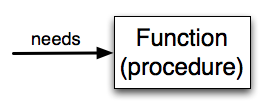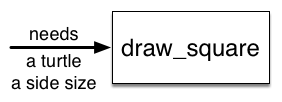# 3. Functions Introduction¶

Quick Overview of Day

Use `for` loops to draw shapes elegantly. Introduce non-fruitful functions in Python. Continue working on a Python turtle graphics assignment, focused on repetition and conditionals.

## 3.1. Warmup Problem¶Draw the shape above. After you have finished drawing your version, share your code with another student. Work together to see if you can come up with a more efficient way to draw this shape!

## 3.2. Functions¶

In Python, a function is a named sequence of statements that belong together. Their primary purpose is to help us organize programs into chunks that match how we think about the solution to the problem.

The syntax for a function definition is the same as it was when we wrote functions in Reeborg:

```def name( parameters ):
statements
```

The one part of this function definition that looks different from our Reeborg investigations is the parameters inside the parentheses. The parameters specify what information, if any, you have to provide in order to use the new function. Another way to say this is that the parameters specify what the function needs to do its work.

There can be any number of statements inside the function, but they have to be indented from the `def`. In the examples in this book, we will use the standard indentation of four spaces.

In a function definition, the keyword in the header is `def`, which is followed by the name of the function and some parameters enclosed in parentheses. The parameter list may be empty, or it may contain any number of parameters separated from one another by commas. In either case, the parentheses are required.

We need to say a bit more about the parameters. In the definition, the parameter list is more specifically known as the formal parameters. This list of names describes those things that the function will need to receive from the user of the function. When you use a function, you provide values to the formal parameters.

The figure below shows this relationship. A function needs certain information to do its work. These values, often called arguments or actual parameters, are passed to the function by the user.This type of diagram is often called a black-box diagram because it only states the requirements from the perspective of the user. The user must know the name of the function and what arguments need to be passed. The details of how the function works are hidden inside the “black-box”.

Suppose we’re working with turtles and a common operation we need is to draw squares. It would make sense if we did not have to duplicate all the steps each time we want to make a square. “Draw a square” can be thought of as an abstraction of a number of smaller steps. We will need to provide two pieces of information for the function to do its work: a turtle to do the drawing and a size for the side of the square. We could represent this using the following black-box diagram.Here is a program containing a function to capture this idea. Give it a try.

This function is named `draw_square`. It has two parameters — one to tell the function which turtle to move around and the other to tell it the size of the square we want drawn. In the function definition they are called `some_turtle` and `side_length` respectively. Make sure you know where the body of the function ends — it depends on the indentation and the blank lines don’t count for this purpose!

docstrings

If the first thing after the function header is a string (some tools insist that it must be a triple-quoted string), it is called a docstring and gets special treatment in Python and in some of the programming tools.

Another way to retrieve this information is to use the interactive interpreter, and enter the expression `<function_name>.__doc__`, which will retrieve the docstring for the function. So the string you write as documentation at the start of a function is retrievable by python tools at runtime. This is different from comments in your code, which are completely eliminated when the program is parsed.

By convention, Python programmers use docstrings for the key documentation of their functions.

Defining a new function does not make the function run. To do that we need a function call (also known as a function invocation). We’ve already seen how to call some built-in functions like `print`, `range` and `int`. Function calls contain the name of the function to be executed followed by a list of values in parentheses, called arguments, which are assigned to the parameters in the function definition. So in the last line of the program, we call the function, and pass `alex` as the turtle to be manipulated, and 50 as the size of the square we want.

Once we’ve defined a function, we can call it as often as we like and its statements will be executed each time we call it. In this case, we could use it to get one of our turtles to draw a square and then we can move the turtle and have it draw a different square in a different location. Note that we lift the tail so that when `alex` moves there is no trace. We put the tail back down before drawing the next square. Make sure you can identify both calls of the `draw_square` function.

In the next example, we’ve changed the `draw_square` function a little and we get `tess` to draw 15 squares with some variations. Once the function has been defined, we can call it as many times as we like with whatever actual parameters we like.

Warning

Even if a function call needs no arguments, the parentheses `( )` after the function name are required. This can lead to a difficult bug: A function name without the parenthesis is a legal expression referring to the function; for example, `print` and `alex.penup`, but they do not call the associated functions.

## 3.3. Practice Problems¶

### 3.3.1. Cross¶Convert the code you wrote at the start of today’s class into a function. You should be able to call something like `draw_cross(some_turtle, side_length)`.

### 3.3.2. Hollow C¶Look back to the code you made when you drew a hollow c last class. Adapt your code by creating a function called `draw_c(a_turtle, longest_side_length, width_of_c)`. You should be able to draw a hollow c by calling the function with something like `draw_c(tess, 150, 25)`.

## 3.4. Turtle Graphics Assignment¶

Use the rest of this class time to keep working on your current Python assignment (possibly a turtle graphics drawing, with a focus on looping and conditionals).

Next Section - 4. Turtle Drawing Practice